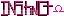-=+=- -=+=- -=+=- -=+=- -=+=- -=+=- -=+=- -=+=- -=+=- -=+=- -=+=- -=+=- -=+=- -=+=- -=+=- -=+=- -=+=- -=+=- -=+=- -=+=- -=+=- -=+=- -=+=- -=+=- -=+=- -=+=- -=+=- -=+=- -=+=- -=+=- (c) WidthPadding Industries 1987 0|523|0 -=+=- -=+=- -=+=- -=+=- -=+=- -=+=- -=+=- -=+=- -=+=- -=+=- -=+=- -=+=- -=+=- -=+=- -=+=- -=+=- -=+=- -=+=- -=+=- -=+=- -=+=- -=+=- -=+=- -=+=- -=+=- -=+=- -=+=- -=+=- -=+=- -=+=-
SoCoder -> Snippet Home -> Variables

PhoenixCreated : 29 June 2009

Language : Monkey

### Fast inverse square root

Found this while browsing about the net, and I thought it could be useful (or at least an interesting read). Lengthy explanation, for the mathematically inclined.

### Comments

Monday, 29 June 2009, 16:19
SchererererThis is really interesting; I skimmed through the research paper, probably give it a closer look a bit later. I've covered Newton's method in the recent pass; probably one of the most important computational approximation methods in existence, but it was very interesting reading how they derived that constant. I'll need to remember this function so I can use it later projects.

Thanks!